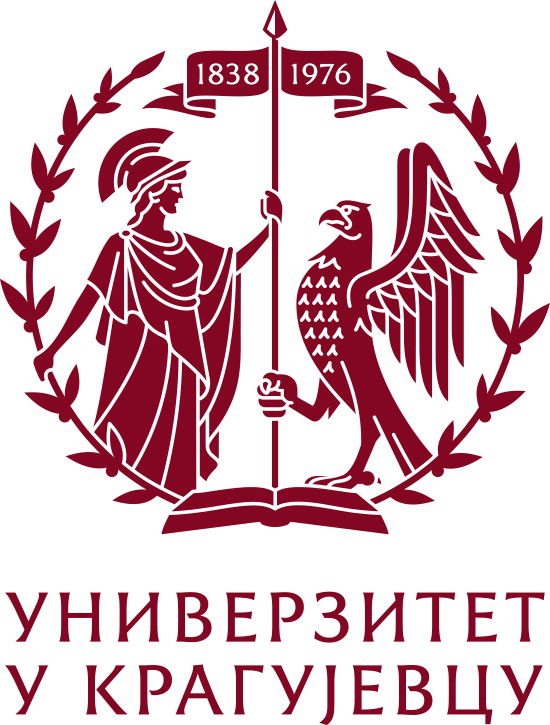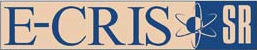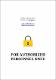Please use this identifier to cite or link to this item: `https://scidar.kg.ac.rs/handle/123456789/11910`
 Title: On Chen ideal submanifolds satisfying some conditions of pseudo-symmetry type Authors: Deszcz, RyszardPetrović-Torgašev, MiroslavaVerstraelen L.Zafindratafa G. Issue Date: 2016 Abstract: © Malaysian Mathematical Sciences Society and Universiti Sains Malaysia 2015. In this paper, we study Chen ideal submanifolds Mn of dimension n in Euclidean spaces 𝔼n+m (n ≥ 4, m ≥ 1) satisfying curvature conditions of pseudo-symmetry type of the form: the difference tensor R · C − C · R is expressed by some Tachibana tensors. Precisely, we consider one of the following three conditions: R·C −C · R is expressed as a linear combination of Q(g, R) and Q(S, R), R·C −C · R is expressed as a linear combination of Q(g, C) and Q(S, C) and R · C − C · R is expressed as a linear combination of Q(g, g∧S) and Q(S, g∧S). We then characterize Chen ideal submanifolds Mn of dimension n in Euclidean spaces 𝔼n+m (n ≥ 4, m ≥ 1) which satisfy one of the following six conditions of pseudo-symmetry type: R·C−C·R and Q(g, R) are linearly dependent, R ·C −C · R and Q(S, R) are linearly dependent, R·C −C · R and Q(g, C) are linearly dependent, R·C −C · R and Q(S, C) are linearly dependent, R · C − C · R and Q(g, g ∧ S) are linearly dependent and R · C − C · R and Q(S, g ∧ S) are linearly dependent. We also prove that the tensors R · R − Q(S, R) and Q(g, C) are linearly dependent at every point of Mn at which its Weyl tensor C is non-zero. URI: https://scidar.kg.ac.rs/handle/123456789/11910 Type: article DOI: 10.1007/s40840-015-0164-7 ISSN: 0126-6705 SCOPUS: 2-s2.0-84953338575 Appears in Collections: Faculty of Science, Kragujevac#### Page views(s)

99

29.86 kBAdobe PDF